# Connection on a principal bundle

(Redirected from Connection form)

## Definition

### Setup

Let$M$ be a differential manifold,$G$ a Lie group acting on$M$, and$\pi: P \to M$ a principal$G$-bundle.

### Definition part

A principal$G$-connection on this principal$G$-bundle is a differential 1-form on$P$ with values in the Lie algebra$\mathfrak{g}$ of$G$ which is$G$-equivariant and reproduces the Lie algebra generators of the fundamental vector fields on$P$.

In other words, it is an element$\omega$ of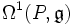$\Omega^1(P,\mathfrak{g})$ such that:

•$Ad(g)(R_g^*\omega) = \omega$ where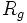$R_g$ denotes right multiplication by$g$. This condition is$G$-equivariance
• If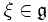$\xi \in \mathfrak{g}$ and$X_\xi$ is the fundamental vector field corresponding to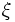$\xi$, then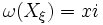$\omega(X_\xi) = xi$ identically on$P$.

## Facts

### Viewing a connection on a vector bundle as a principal connection

Fill this in later

### Transport using principal connections

Fill this in later